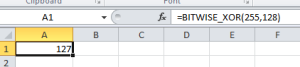The `NOT`, `AND`, `OR` and `XOR` functions in Microsoft Excel are logical functions and not bitwise functions. What this means is that they only return `TRUE` or `FALSE` based on the input expressions given.

In Microsoft Excel 2013, Microsoft has released new functions that work on the bit level: `BITNOT`, `BITAND`, `BITOR` and `BITNOT`. But these functions are not available in earlier versions of Excel (eg. Excel 2007 or Excel 2010).

If we want to have similar functionality in Excel 2010 or earlier, we will need to implement the functions in Excel VBA instead. To do that, open the Visual Basic editor by pressing `ALT-F11`. Once you are in the Excel VBA, create a new `Module` from the `Insert` Menu and copy the following lines of Visual Basic code to the Module.

```Public Function BITWISE_XOR(x As Long, y As Long)
BITWISE_XOR = x Xor y
End Function

Public Function BITWISE_NOT(x As Long)
BITWISE_NOT = Not x
End Function

Public Function BITWISE_AND(x As Long, y As Long)
BITWISE_AND = x And y
End Function

Public Function BITWISE_OR(x As Long, y As Long)
BITWISE_OR = x Or y
End Function
```

Save the VBA Module and exit from the editor. Then make sure that you save the original file as `Excel Macro-Enabled Workbook` and not as just an Excel Workbook, else the above VBA module will not be saved.Now, we are able to use the new custom Excel bitwise functions. The screenshot above shows an example on how the `BITWISE_XOR` function can be used.

### Mohamed Ibrahimibrahim = { interested_in(unix, linux, android, open_source, reverse_engineering); coding(c, shell, php, python, java, javascript, nodejs, react); plays_on(xbox, ps4); linux_desktop_user(true); }